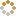﻿ Ningbo University of Technology Online Judge :: NBUT 2015 Timed NOJ Training #004 :: XorSum
• ### [A] XorSum

• 时间限制: 2000 ms　内存限制: 65535 K
• 问题描述
• You are Given n(1 <= n <= 100) integers (each number no more than 100 and no less than 0), now you must choose m numbers and the xor value is maximum.

• 输入
• Input starts with an integer T denoting the number of test case.
For each test case, first line contains n and m, the second line contains n integers.
• 输出
• For each test case, firstly print "Case X: " where X is the case number and then print the maximum xor value.
• 样例输入
• ```2
4 1
1 2 3 4
3 3
1 1 1```
• 样例输出
• ```4
1```
• 提示
• `无`
• 来源
• `Alex@NBUT`
• 操作
﻿